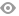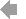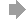﻿ Page 8 - MATINF Nr. 6
Basic HTML VersionView Full Version
Page 8 - MATINF Nr. 6
P. 8

``````8                                                                                E.A. Jos´e Garc´ıa

Fig. 2: A bicentric quadrilateral ABCD.

Proof. Since a + c = b + d in a bicentric quadrilateral, the formula (3) reduces to

cos 2  =         .

Similarly we can get sin 2 α  =  bc  . Now, following the same steps as in Brahmagupta’s
formula
◦
ad sin α   bc sin (180 − α)
∆ 2 =         +
2               2
ad sin α   bc sin α
=          +
2          2
α     α
= sin   cos (ad + bc)
2     2
Ê         Ê
√
=    abcd.

The following theorem generalizes Theorem 1 for a general convex quadrilateral.

Theorem 5. Let a, b, c, d be the sides of a general convex quadrilateral, s is the semiperimeter,
and α and γ are opposite angles, then

α           γ
ad sin 2  + bc cos 2  = (s − a)(s − d)                          (5)
2           2

and
γ           α
bc sin 2  + ad cos 2  = (s − b)(s − c).                          (6)
2            2

Proof. By the Law of Cosines,

2
2
2
2
a + d − 2ad cos α = b + c − 2bc cos γ.``````3   4   5   6   7   8   9   10   11   12   13